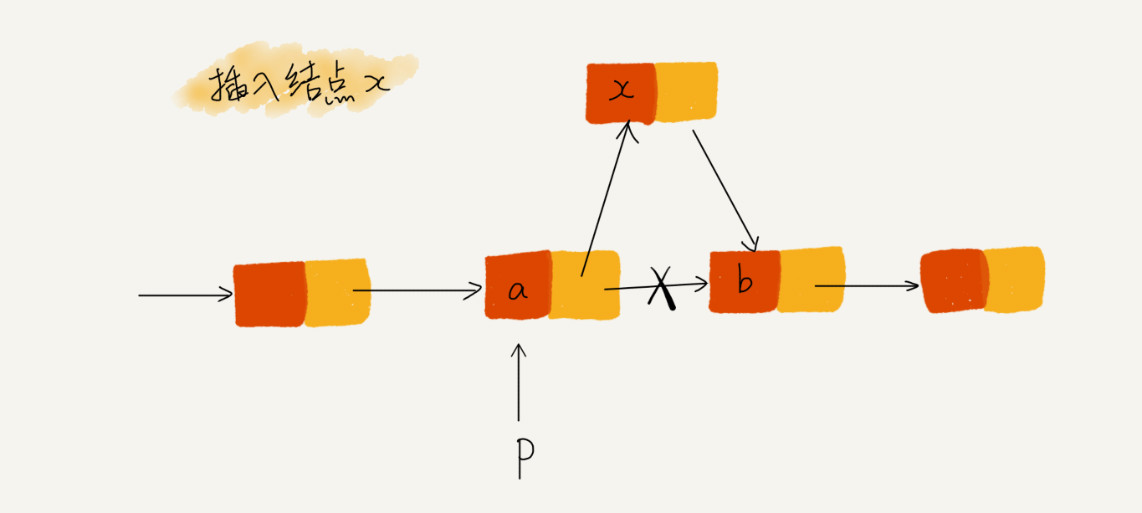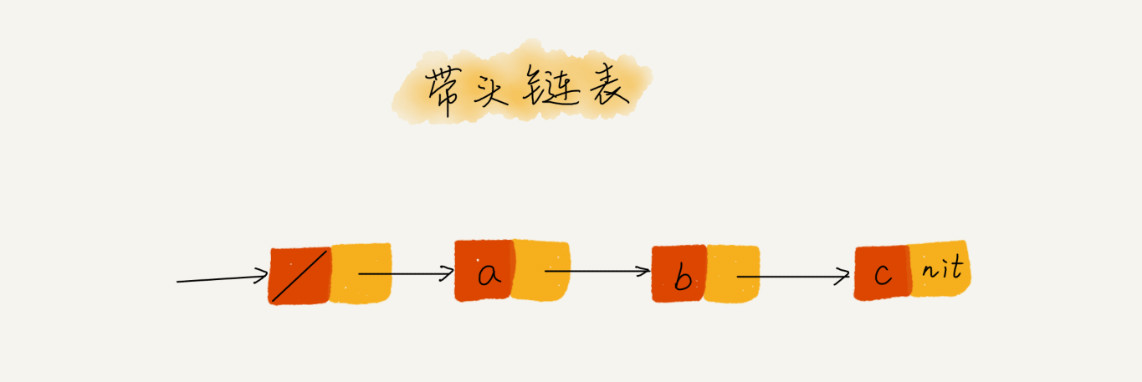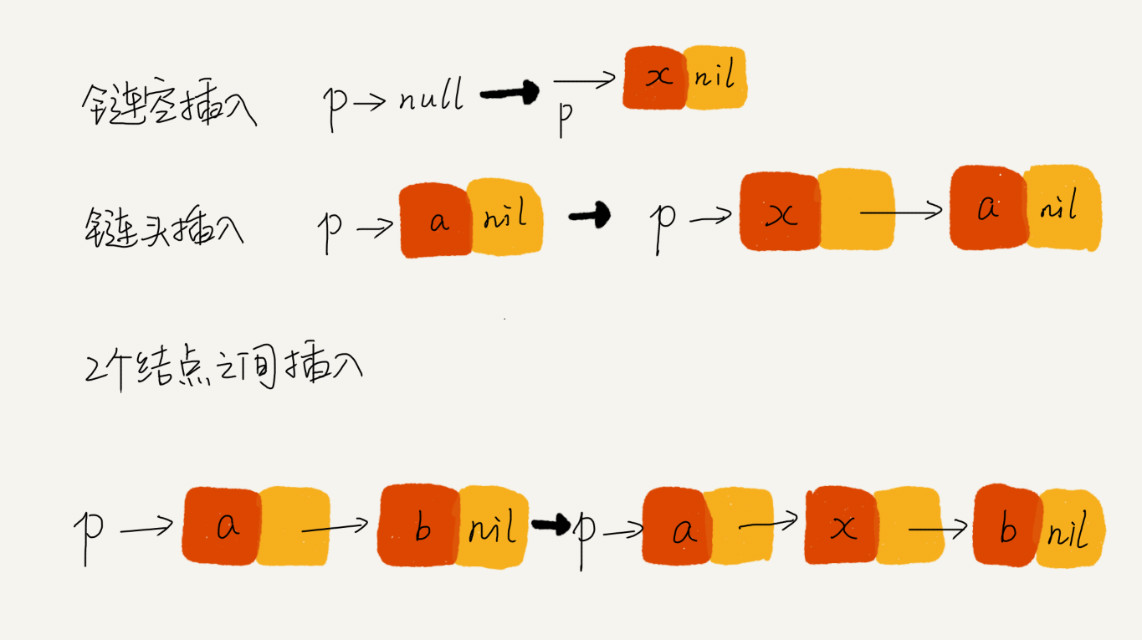## 技巧二：警惕指针丢失和内存泄漏``````p->next = x;  // 将 p 的 next 指针指向 x 结点；
x->next = p->next;  // 将 x 的结点的 next 指针指向 b 结点；``````

`p->next` 指针在完成第一步操作之后，已经不再指向结点 `b` 了，而是指向结点 `x`。第 `2` 行代码相当于将 `x` 赋值给 `x->next`，自己指向自己。因此，整个链表也就断成了两半，从结点 `b` 往后的所有结点都无法访问到了。

## 技巧三：利用哨兵简化实现难度

``````new_node->next = p->next;
p->next = new_node;``````

``````if (head == null) {
}``````

``p->next = p->next->next;``

``````if (head->next == null) {
}````````````// 在数组 a 中，查找 key，返回 key 所在的位置
// 其中，n 表示数组 a 的长度
int find(char* a, int n, char key) {
// 边界条件处理，如果 a 为空，或者 n<=0，说明数组中没有数据，就不用 while 循环比较了
if(a == null || n <= 0) {
return -1;
}

int i = 0;
// 这里有两个比较操作：i<n 和 a[i]==key.
while (i < n) {
if (a[i] == key) {
return i;
}
++i;
}

return -1;
}``````

``````// 在数组 a 中，查找 key，返回 key 所在的位置
// 其中，n 表示数组 a 的长度
// 我举 2 个例子，你可以拿例子走一下代码
// a = {4, 2, 3, 5, 9, 6}  n=6 key = 7
// a = {4, 2, 3, 5, 9, 6}  n=6 key = 6
int find(char* a, int n, char key) {
if(a == null || n <= 0) {
return -1;
}

// 这里因为要将 a[n-1] 的值替换成 key，所以要特殊处理这个值
if (a[n-1] == key) {
return n-1;
}

// 把 a[n-1] 的值临时保存在变量 tmp 中，以便之后恢复。tmp=6。
// 之所以这样做的目的是：希望 find() 代码不要改变 a 数组中的内容
char tmp = a[n-1];
// 把 key 的值放到 a[n-1] 中，此时 a = {4, 2, 3, 5, 9, 7}
a[n-1] = key;

int i = 0;
// while 循环比起代码一，少了 i<n 这个比较操作
while (a[i] != key) {
++i;
}

// 恢复 a[n-1] 原来的值, 此时 a= {4, 2, 3, 5, 9, 6}
a[n-1] = tmp;

if (i == n-1) {
// 如果 i == n-1 说明，在 0...n-2 之间都没有 key，所以返回 -1
return -1;
} else {
// 否则，返回 i，就是等于 key 值的元素的下标
return i;
}
}``````

## 技巧四：重点留意边界条件处理

• 如果链表为空时，代码是否能正常工作？

• 如果链表只包含一个结点时，代码是否能正常工作？

• 如果链表只包含两个结点时，代码是否能正常工作？

• 代码逻辑在处理头结点和尾结点的时候，是否能正常工作？

## 技巧五：举例画图，辅助思考## 技巧六：多写多练，没有捷径

• 单链表反转

• 链表中环的检测

• 两个有序的链表合并

• 删除链表倒数第 n 个结点

• 求链表的中间结点